#### Residual-Based Estimators

The GLIMMIX procedure can compute the classical sandwich estimator of the covariance matrix of the fixed effects, as well as several bias-adjusted estimators. This requires that the model is either an (overdispersed) GLM or a GLMM that can be processed by subjects (see the section Processing by Subjects).

Consider a statistical model of the form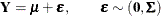The general expression of a sandwich covariance estimator is then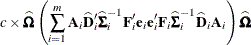where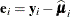,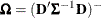.

For a GLMM estimated by one of the pseudo-likelihood techniques that involve linearization, you can make the following substitutions: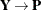,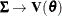,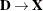,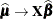. These matrices are defined in the section Pseudo-likelihood Estimation Based on Linearization.

The various estimators computed by the GLIMMIX procedure differ in the choice of the constant c and the matrices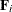and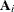. You obtain the classical estimator, for example, with c = 1, and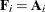equal to the identity matrix.

The EMPIRICAL=ROOT estimator of Kauermann and Carroll (2001) is based on the approximation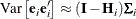where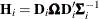. The EMPIRICAL=FIRORES estimator is based on the approximation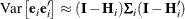of Mancl and DeRouen (2001). Finally, the EMPIRICAL=FIROEEQ estimator is based on approximating an unbiased estimating equation (Fay and Graubard, 2001). For this estimator,is a diagonal matrix with entries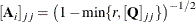where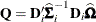. The optional number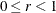is chosen to provide an upper bound on the correction factor. For r = 0, the classical sandwich estimator results. PROC GLIMMIX chooses as default value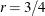. The diagonal entries ofare then no greater than 2.

Table 41.24 summarizes the components of the computation for the GLMM based on linearization, where m denotes the number of subjects and k is the rank of.

Table 41.24: Empirical Covariance Estimators for a Linearized GLMM

EMPIRICAL=

cCLASSICAL

1DF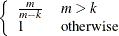ROOT

1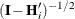FIRORES

1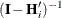FIROEEQ(r)

1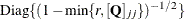Computation of an empirical variance estimator requires that the data can be processed by independent sampling units. This is always the case in GLMs. In this case, m equals the sum of all frequencies. In GLMMs, the empirical estimators require that the data consist of multiple subjects. In that case, m equals the number of subjects as per the Dimensions table. The following section discusses how the GLIMMIX procedure determines whether the data can be processed by subjects. The section GLM Mode or GLMM Mode explains how PROC GLIMMIX determines whether a model is fit in GLM mode or in GLMM mode.